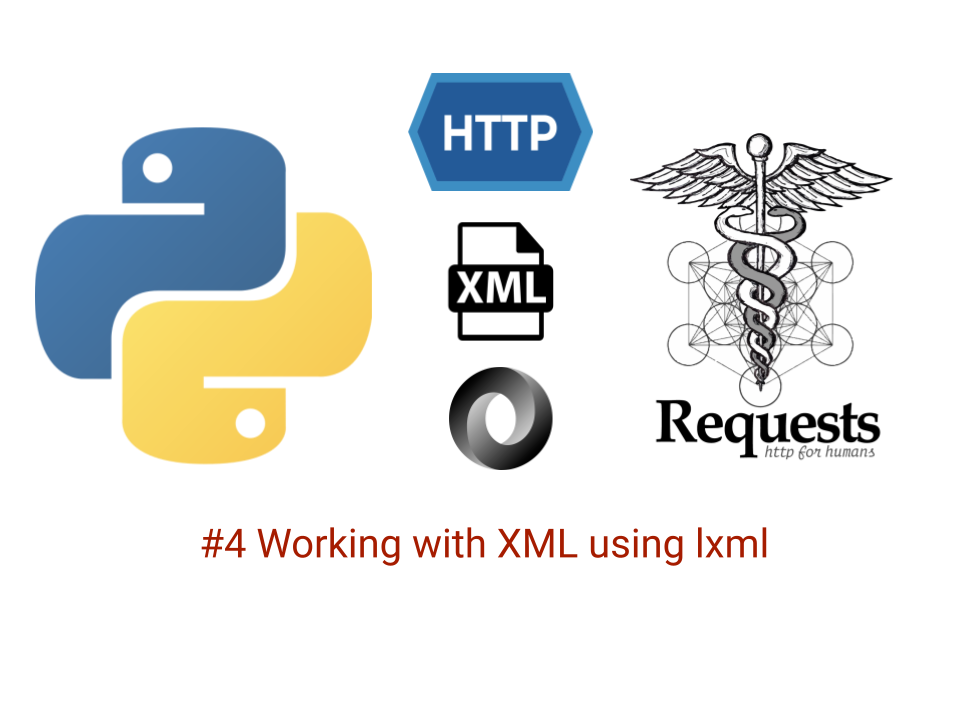# Python API test automation framework (Part 4) Working with XML using python lxmlLogos in header image sources: Python, Requests, JSON, HTTP

This is fourth post in a series on how to build an API framework using python.

You can read previous parts below:

Any API framework would be incomplete without having the ability to deal with XML responses and requests.

You might primarily need this if you are automating a SOAP (Simple object access protocol) based services in your project or if you choose to use XML as a data format for configuration, test data and what not.

Though JSON, YAML are probably a more reasonable bet for this. XML is still quite a popular data format

Let’s see how can we work with XML

## Introducing lxml

To work with XML in python, we would make use of the popular and powerful lxml library which is very useful for dealing with XML and is a wrapper over C libraries like libxml2 and libxslt while retaining the simplicity of a native Python API

Let’s get started.

To set up, Ensure you have installed it in your pipenv using:

``````pipenv install lxml
``````

## An example

Let’s consider we have to verify the XML response from an API that returns (the infamous) Covid data for the day with an overall world summary and a country wide summary breakup.

I’ve created a dummy service called covid_tracker.py which is a python flask API to return a canned and static XML response

To ensure the service is running, execute below commands

``````# cd to dir
cd people-api
# activate pipenv and ensure all dependencies are installed
pipenv shell
pipenv install
# Run the local flask service
python covid_tracker/covid_tracker.py
``````

Below is the cURL for this:

``````curl --location --request GET 'http://localhost:3000/api/v1/summary/latest'
``````

And this would return a response like below:

``````<?xml version="1.0" encoding="UTF-8" ?>
<root>
<status>200</status>
<type>stack</type>
<data>
<summary>
<total_cases>69169558</total_cases>
<active_cases>19895522</active_cases>
<deaths>1574941</deaths>
<recovered>47699103</recovered>
<critical>104419</critical>
<tested>1003760026</tested>
<death_ratio>0.022769279514551762</death_ratio>
<recovery_ratio>0.6895967587359746</recovery_ratio>
</summary>
<change>
<total_cases>653173</total_cases>
<active_cases>142334</active_cases>
<deaths>12042</deaths>
<recovered>498799</recovered>
<critical>164</critical>
<tested>13146244</tested>
<death_ratio>-0.00004130805512226471</death_ratio>
<recovery_ratio>0.0007060065458233122</recovery_ratio>
</change>
<generated_on>1607547603</generated_on>
<regions>
<usa>
<name>USA</name>
<iso3166a2>US</iso3166a2>
<iso3166a3>USA</iso3166a3>
<iso3166numeric></iso3166numeric>
<total_cases>15740193</total_cases>
<active_cases>6277786</active_cases>
<deaths>295403</deaths>
<recovered>9167004</recovered>
<critical>26975</critical>
<tested>212565283</tested>
<death_ratio>0.018767431886000382</death_ratio>
<recovery_ratio>0.5823946377277585</recovery_ratio>
<change>
<total_cases>222261</total_cases>
<active_cases>84705</active_cases>
<deaths>2828</deaths>
<recovered>134728</recovered>
<death_ratio>-0.00008656231889753868</death_ratio>
<recovery_ratio>0.0003405341268405415</recovery_ratio>
</change>
</usa>
``````

Let’s say, hypothetically we want to check that this API returns a valid no greater than a million of total worldwide cases and write a test for this.

Below is a test that achieves this.

``````import requests
from assertpy import assert_that
from lxml import etree

from config import COVID_TRACKER_HOST
from utils.print_helpers import pretty_print

def test_covid_cases_have_crossed_a_million():
response = requests.get(f'{COVID_TRACKER_HOST}/api/v1/summary/latest')

response_xml = response.text
xml_tree = etree.fromstring(bytes(response_xml, encoding='utf8'))

# use .xpath on xml_tree object to evaluate the expression
total_cases = xml_tree.xpath("//data/summary/total_cases").text
assert_that(int(total_cases)).is_greater_than(1000000)
``````

Let’s break it down and understand whats happening here.

We make an HTTP Get call to our GET API `/api/v1/summary/latest` and get the response XML in text format.

``````response = requests.get(f'{COVID_TRACKER_HOST}/api/v1/summary/latest')
response_xml = response.text
``````

Next, to make use of this XML response, we need to deserialize (i.e. string to python object) it into a ElementTree object

Element tree belongs to the lxml library.

This can be done with below:

``````tree = etree.fromstring(bytes(response_xml, encoding='utf8'))
``````

📝 Its important to provide `fromstring()` data in bytes format with UTF-8 encoding since without that it would give error like: ValueError: Unicode strings with encoding declaration are not supported. Please use bytes input or XML fragments without declaration.

tree is now an object representation of the XML string and we can then use `node.xpath('<your_xpath_expression>')` to get the required node which we want to process.

In our current case we want the `total_cases` node under the `summary` section.

We can get that using relative XPath expression as follows. If you are unfamiliar with XPath syntax, you refer to this tutorial on w3schools.com

``````total_cases = tree.xpath("//data/summary/total_cases").text
``````

To get the text in the first node we use `.text` property

And finally now that we have the desired node, we could assert as follows

``````assert_that(int(total_cases)).is_greater_than(1000000)
``````

## Another way to work with XPath using lxml

There is another way to make use of XPath wherein you can specify the expression upfront and then use it as needed.

Let’s say we want to assert that the total cases worldwide is greater than the total of cases across countries.

Below is the test, we could write for this:

``````def test_overall_covid_cases_match_sum_of_total_cases_by_country():
response = requests.get(f'{COVID_TRACKER_HOST}/api/v1/summary/latest')

response_xml = response.text
xml_tree = etree.fromstring(bytes(response_xml, encoding='utf8'))

overall_cases = int(xml_tree.xpath("//data/summary/total_cases").text)
# Another way to specify XPath first and then use to evaluate
# on an XML tree
search_for = etree.XPath("//data//regions//total_cases")
cases_by_country = 0
for region in search_for(xml_tree):
cases_by_country += int(region.text)

assert_that(overall_cases).is_greater_than(cases_by_country)
``````

First few lines should be familiar now, Notice we use:

``````search_for = etree.XPath("//data//regions//total_cases")
``````

Which gives us an XPath object but does not evaluate it as that point itself.

We make use of it to get a list of elements from the XPath and then use a loop to get the total for that specific region

``````cases_by_country = 0
for region in search_for(xml_tree):
cases_by_country += int(region.text)
``````

And finally we can assert:

``````assert_that(overall_cases).is_greater_than(cases_by_country)
``````

When I run this test, I can see it fail:

``````>       assert_that(overall_cases).is_greater_than(cases_by_country)
E       AssertionError: Expected <69169558> to be greater than <69822731>, but was not.
``````

Which means that there is data mismatch bug 🐛 in this data set

## Conclusion

There are many other use cases which the lxml library can support. Discussing these here would result in a very long post. I would encourage you to get into the very well written lxml docs when in doubt, for more details on your specific use cases.

You can find the complete code for this course on Github at automationhacks/course-api-framework-python

Tags:

Categories:

Updated: